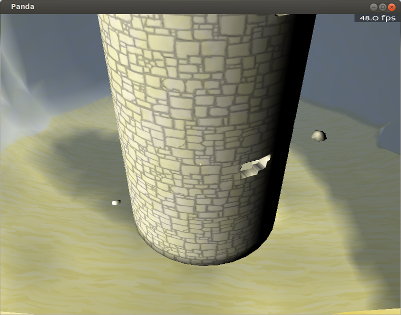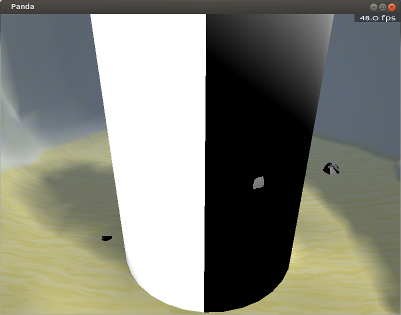# Baby's First Normal Mapping Shader

I’m currently attempting to implement a normal mapping shader. Based on what I’ve read, my shader looks as though it should do the job–but it doesn’t, and I’m really not seeing where I’m going wrong.

The shader in this post is reported by the poster to work, and has been one of the references that I’ve used.

Below is what I have thus far; I’m sorry to dump so much code, but I’m really not sure of where I’m going wrong…It might be worth noting that my normal map is intended to be tiled, as it corresponds to a tiled diffuse map (specifically, stone wall-blocks). The diffuse map seems to tile correctly, so I would expect the normal map to do so as well. The model does seem to have tangents and binormals, as exported via YABEE.

Actual code:
(There are a few bits in here that aren’t used by the normal-mapping code, but they shouldn’t take up much space.)

``````void vshader(
in float4 vtx_texcoord0: TEXCOORD0,
in float4 vtx_position: POSITION,
in float3 vtx_normal: NORMAL,
in float3 vtx_tangent0,
in float3 vtx_binormal0,
in float4 vtx_color: COLOR,
in uniform float4x4 mat_modelproj,
in uniform float4x4 mstrans_world,
out float4 l_texcoord0: TEXCOORD0,
out float4 l_position: POSITION,
out float4 l_color: COLOR,
out float3 l_normal,
out float3 l_tangent0,
out float3 l_binormal0,
out float4 l_screenVtxPos
)
{
l_position = mul(mat_modelproj, vtx_position);
l_texcoord0 = vtx_texcoord0;
l_normal = vtx_normal.xyz;
l_tangent0 = vtx_tangent0;
l_binormal0 = -vtx_binormal0;
l_screenVtxPos = mul(mat_modelproj, vtx_position);
l_color = vtx_color;
}

uniform sampler2D tex_0,
in float4 l_texcoord0: TEXCOORD0,
in float3 l_normal,
in float3 l_tangent0,
in float3 l_binormal0,
in float4 l_screenVtxPos,
in float4 l_color: COLOR,
in uniform float4x4 mstrans_world,
in uniform sampler2D tex_normal,
out float4 o_color: COLOR0
)
{
float dist = abs(l_screenVtxPos.z);
o_color = tex2D(tex_0, l_texcoord0.xy);
float3 normal = normalMap(l_normal, l_tangent0, l_binormal0, tex_normal, l_texcoord0);
//  This lighting is temporary; my main lighting shader is a little more
// complex, and I wanted to remove that complexity while
// attempting to fix the normal-mapping.
float4 lightDir4 = float4(-1, -1, 1, 0);
float3 lightDir = normalize((mul(mstrans_world, lightDir4)).xyz);
o_color = o_color*dot(normal, lightDir);
}``````

The normal-mapping function:

``````float3 normalMap(
in float3 l_normal,
in float3 l_tangent0,
in float3 l_binormal0,
in uniform sampler2D tex_normal,
in float4 l_texcoord0)
{
float4 normalOffsetVec = tex2D(tex_normal, l_texcoord0.xy)*2.0 - 1.0;
float3 right = l_binormal0*normalOffsetVec.x;
float3 up = l_tangent0*normalOffsetVec.y;
float3 norm = l_normal*normalOffsetVec.z;

float3 result = normalize(up + right + norm);

return result;
}``````

Use of the shader in Python script:

``````        #  The shader is only intended to be applied to a part
# been applied to a NodePath above this, with the
# intention being that this shader replaces that.
tower = geometry.find("**/Cylinder.001")
if not tower.isEmpty():
print "found tower"

Does anyone see where I’m going wrong?Okay, this seems very strange…

I’ve encountered and anomaly; it may or may not be related to the above–I really don’t know what’s going on here… o_0

In experimenting in the hopes of finding a solution to the problem described in the post above, I stumbled on something else. Something seems to be wrong with my calculation of lighting from a normal and light direction. Consider the code below: it should, given its hard-coded light direction, the surface normal, and a base colour, produce an appropriately-shaded colour. When that base colour is sampled from my texture, it seems to work as expected. However, If I replace that sampling with the static value “float4(1, 1, 1, 1)” (pure white–I am correct in that, am I not?) I end up with a strange discontinuity that seems to show up at UV-seams.

To illustrate:

The standard case:

``````void fshader(
uniform sampler2D tex_0,
in float4 l_texcoord0: TEXCOORD0,
in float3 l_normal,
in float4 l_screenVtxPos,
in float4 l_color: COLOR,
in uniform float4x4 mstrans_world,
out float4 o_color: COLOR0
)
{
o_color = tex2D(tex_0, l_texcoord0.xy);
float3 normal = l_normal;
float4 lightDir4 = float4(0.704, 0.704, 0.704, 0);
float3 lightDir = mul(mstrans_world, lightDir4).xyz;
normalize(lightDir);
float light = max(0, dot(l_normal, lightDir));
o_color = o_color*light;
}``````

The result:In the anomalous case, the only change is to the line that samples the texture, I believe:

``    o_color = float4(1, 1, 1, 1);//tex2D(tex_0, l_texcoord0.xy);``

The result:bump Does no-one have any insight into this?

I think it might be a good idea to start off with a working shader, for example a dumped shader generated by the auto-shader.
Something like this:

``````//Cg
/* Generated shader for render state 09DE34E8:
LightAttrib:on
render/dlight
TextureAttrib:on default:215 Tex2:215-normal
*/
in float4 vtx_texcoord0 : TEXCOORD0,
out float4 l_texcoord0 : TEXCOORD0,
in float4 vtx_texcoord1 : TEXCOORD1,
out float4 l_texcoord1 : TEXCOORD1,
uniform float4x4 trans_model_to_view,
out float4 l_eye_position : TEXCOORD2,
uniform float4x4 tpose_view_to_model,
out float4 l_eye_normal : TEXCOORD3,
in float4 vtx_normal : TEXCOORD2,
in float4 vtx_tangent1 : TEXCOORD3,
in float4 vtx_binormal1 : TEXCOORD4,
out float4 l_tangent : TEXCOORD4,
out float4 l_binormal : TEXCOORD5,
float4 vtx_position : POSITION,
out float4 l_position : POSITION,
uniform float4x4 mat_modelproj
) {
l_position = mul(mat_modelproj, vtx_position);
l_eye_position = mul(trans_model_to_view, vtx_position);
l_eye_normal.xyz = mul((float3x3)tpose_view_to_model, vtx_normal.xyz);
l_eye_normal.w = 0;
l_texcoord0 = vtx_texcoord0;
l_texcoord1 = vtx_texcoord1;
l_tangent.xyz = mul((float3x3)tpose_view_to_model, vtx_tangent1.xyz);
l_tangent.w = 0;
l_binormal.xyz = mul((float3x3)tpose_view_to_model, -vtx_binormal1.xyz);
l_binormal.w = 0;
}

in float4 l_eye_position : TEXCOORD2,
in float4 l_eye_normal : TEXCOORD3,
uniform sampler2D tex_0,
in float4 l_texcoord0 : TEXCOORD0,
uniform sampler2D tex_1,
in float4 l_texcoord1 : TEXCOORD1,
in float3 l_tangent : TEXCOORD4,
in float3 l_binormal : TEXCOORD5,
uniform float4x4 dlight_dlight0_rel_view,
out float4 o_color : COLOR0,
uniform float4 attr_color,
uniform float4 attr_colorscale
) {
float4 result;
// Fetch all textures.
float4 tex0 = tex2D(tex_0, l_texcoord0.xy);
float4 tex1 = tex2D(tex_1, l_texcoord1.xy);
// Translate tangent-space normal in map to view-space.
float3 tsnormal = ((float3)tex1 * 2) - 1;
l_eye_normal.xyz *= tsnormal.z;
l_eye_normal.xyz += l_tangent * tsnormal.x;
l_eye_normal.xyz += l_binormal * tsnormal.y;
// Correct the surface normal for interpolation effects
l_eye_normal.xyz = normalize(l_eye_normal.xyz);
// Begin view-space light calculations
float ldist,lattenv,langle;
float4 lcolor,lspec,lvec,lpoint,latten,ldir,leye,lhalf;
float4 tot_diffuse = float4(0,0,0,0);
// Directional Light 0
lcolor = dlight_dlight0_rel_view;
lspec  = dlight_dlight0_rel_view;
lvec   = dlight_dlight0_rel_view;
lcolor *= saturate(dot(l_eye_normal.xyz, lvec.xyz));
tot_diffuse += lcolor;
// Begin view-space light summation
result = float4(0,0,0,0);
result += tot_diffuse;
result = saturate(result);
// End view-space light calculations
result.rgb *= tex0.rgb;
result *= attr_colorscale;
o_color = result * 1.000001;
}``````

the shaders inputs needed for this one is just the light:

``````dlight = DirectionalLight('dlight')
dlight.setColor(VBase4(1, 1, 1, 1))
dlnp = render.attachNewNode(dlight)

For other tips… you can use the normal vector xyz as a rgb color for debuging.

Ah, thank you! Your post seems to have helped me to fix my shader!There seem to have been two problems:

1. My parameters for the normal, both as output from the vertex shader and as input into the fragment shader had no " : <some_ID>" suffix; changing this to use " : TEXCOORD3", as shown in the example, seems to have done the trick.
• If I may ask, why is this? What does that identifier do that causes it to work when a simply “float3” alone doesn’t?
1. I was apparently parameterising my normal-map texture incorrectly. Simply put, I’m adding it as a shader input, rather than as a normally-added texture–and was naming it “tex_normal”, which seemed to be a problem. Renaming it to “texNormal” seems to have fixed this issue.

The “normal vector as colour” trick is quite a good one, and proved helpful, I do think–thank you. It also produced some wonderfully trippy visuals.1. You have to bind it to a specific register for it to pass correctly between the vertex and fragment shader. Supposedly there is by-name binding when semantics aren’t specified, but it doesn’t always work well in Cg.

2. Panda has special handling for the tex_ prefix - when you specify tex_0, for instance, it automatically binds to the texture specified by the first texture stage. When you specified tex_normal, presumably it failed to convert “normal” to integer and took the first texture as a fallback.

Ahh, fair enough and thank you! The explanation is appreciated.